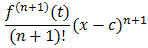MathCS.org - Real Analysis: Proposition 8.4.8: Lagrange's Version of Taylor Remainder

#Interactive Real Analysis

Next | Previous | Glossary | Map

## 8.4. Taylor Series

### Proposition 8.4.8: Lagrange's Version of Taylor Remainder

Suppose fCn+1([a, b]), i.e. f is (n+1)-times continuously differentiable on [a, b]. Then, for c[a,b] we have:
f(x) =where
R(n+1)(x) =for some t between x and c.

Taylor's theorem gives us this result, except for the remainder, which is different. What we have to show, therefore, is that Taylor's remainder and the Lagrange remainder are really the same. In other words we need to show that:

Rn+1(x) = 1/n!(x-t)nf (n+1)(t) dt =But this follows directly from the mean value theorem for integration (make sure to provide the details).Next | Previous | Glossary | Map